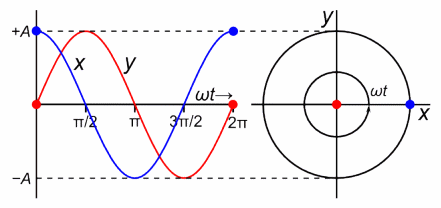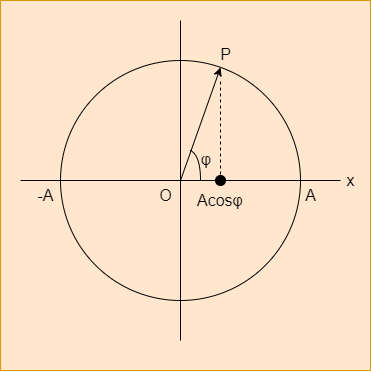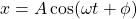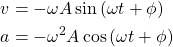# Physics - Classical Mechanics - Simple Harmonic Motion and Reference Circle

in STEMGeeks5 months ago[Image1]

## Introduction

Hey it's a me again @drifter1!

Today we continue with Physics, and more specifically the branch of "Classical Mechanics" in order to cover Simple Harmonic Motion and the Reference Circle.

So, without further ado, let's get straight into it!

## SHM and Smooth Circular Motion

A Smooth Harmonic Motion model can also be constructed from Uniform Circular Motion, as the mathematical equations that describe SHM relate to the concepts of circular motion.

Starting off from a circular motion with a radius A (amplitude) and angular frequency ω, the corresponding SHM is the projection of the so called rotating phase vector on the horizontal axis (or diameter). The projection is thus equal to the cosinus of each individual angle (or phase) φ, times the amplitude A, as shown below.If at t = 0, the angular position is φ, as it rotates this angle (or phase) changes by ωt, meaning that the angular position at any t is equal to φ + ωt. The projection to the horizontal axis is then equal to:which is the SHM equation for displacement that we proved last time!

## Reference Circle

The diagram shown in the previous section is the so called Reference Circle. As the point P moves anti-clockwise with an angular frequency of ω, the vector from the center O to this point forms an angle of φ to the horizontal axis. Projecting this rotating phase vector to the horizontal axis yields the position of the corresponding SHM.

Additionally, the horizontal components of the velocity and acceleration of this circular motion give us the velocity and acceleration fo the corresponding SHM. The velocity of point P is equal to ωΑ, whilst its acceleration is ωA2, giving us the equations:that where proven last time.

Some key phase angles and the corresponding displacements are:

Phase (φ)Displacement (x)
0A
π / 6 (30°)~ 0.86A
π / 4 (45°)~ 0.7A
π / 3 (60°)0.5A
π / 2 (90°)0
π (180°)-A
3π / 2 (270°)0

Now we are ready for full-on examples, which will come next time!

## Final words | Next up

And this is actually it for today's post!

Next time we will get into exercises on the topic of Simple Harmonic Motion...

See ya!Keep on drifting!

Posted with STEMGeeks

Sort:Thanks for your contribution to the STEMsocial community. Feel free to join us on discord to get to know the rest of us!

Please consider delegating to the @stemsocial account (85% of the curation rewards are returned).

You may also include @stemsocial as a beneficiary of the rewards of this post to get a stronger support.

Dear @drifter1,

Do you mind supporting the HiveBuzz proposal for 2022 so our team can continue its work next year?
You can do it on Peakd, ecency, Hive.blog or using HiveSigner.
https://peakd.com/me/proposals/199

We wish you a Happy New Year!